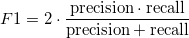# Precision, Recall and F1 score (information retrieval)

Suppose we are playing a word game: I think a few words and you have to guess them.
As usually you will ask me some questions, identify the subject and try to guess. A possible end of the game is:

• I think: rabbit, carrot, orange.
• You try: dog, carrot, peach, orange.

We can define 2 quantities to synthesize how good you are as this game:

• precision: is the number of correct guess over the number of tries = 2/4
• recall: is the number of correct guess over the number of words to guess = 2/3

It’s easy to see that this two numbers are somehow in competition.

If you start reading a vocabulary you will end up having a good recall at the price of a very small precision:

• precision = 3/10.000
• recall = 3/3

On the other side, spending a lot of time investigating for a single word can result in a high precision at the price of a small recall:

• precision = 1/1
• recall = 1/3

We have 2 numbers, Precision and Recall, and we need to chose the strategy to play, so we need some way to merge them in a single value, rank the methods and chose the best one.

The F1 score is a standard way to mix the two numbers in a single score:Let’s compute the F1 score for the tree proposed solutions:

• your method scores F1 = 0.57
• the dictionary method scores F1= 0.0006
• the investigator method scores F1=0.5

So, the best score is the one of your solution!